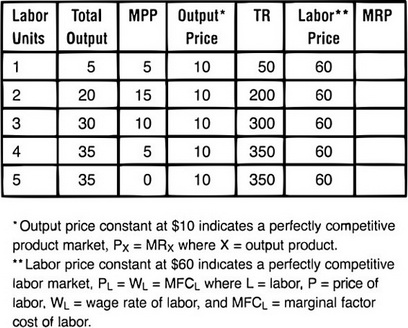# AP Microeconomics Practice Test: Resource Markets with Applications to Labor

### Test Information6 questions7 minutes

1.With the data in the table above, how many units of labor would the employer hire?

2. The basis for the answer in number 1 is

3. Which of the following is not the basis for a wage differential?

4. A monopsonist is identified by one of the following:

5. If for two resources, Labor (L) and Capital (K), the ratios of their marginal physical products are
MPPK/PK = MPPL/PL
the firm should:

6. The backward bending supply curve is characterized by which of the following?